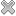Courses / Module

Toggle PrintSTATISTICAL METHODS FOR DATA SCIENCE 1 (R)

Module code: DS863
Credits: 5
Semester: 1
Department: MATHEMATICS AND STATISTICS
International:Overview

This course covers probability and statistical techniques for data analytics and data science. Topics include: Exploratory data analysis and visualisation. Probability basics, independence, Bayes theorem. Probability models for data, including Binomial, Poisson, Exponential and Normal. Confidence intervals and hypothesis testing: one and two samples, paired samples, proportions. Case studies in R.

Lectures and labs as for DS663.Learning OutcomesTeaching & Learning methodsAssessmentRepeat optionsPre-RequisitesTimetable